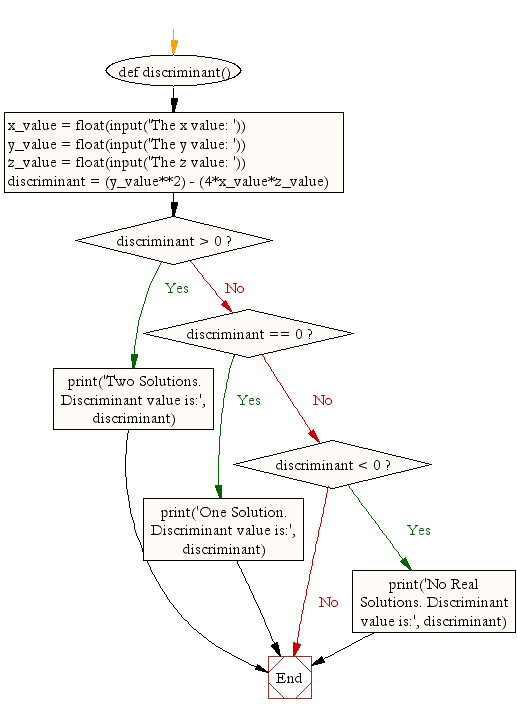﻿ Python Math: Calculate the discriminant value - w3resource# Python Math: Calculate the discriminant value

## Python Math: Exercise-9 with Solution

Write a Python program to calculate the discriminant value.

Sample Solution:-

Python Code:

``````def discriminant():
x_value = float(input('The x value: '))
y_value = float(input('The y value: '))
z_value = float(input('The z value: '))
discriminant = (y_value**2) - (4*x_value*z_value)
if discriminant > 0:
print('Two Solutions. Discriminant value is:', discriminant)
elif discriminant == 0:
print('One Solution. Discriminant value is:', discriminant)
elif discriminant < 0:
print('No Real Solutions. Discriminant value is:', discriminant)

discriminant()
```
```

Sample Output:

```The x value: 4
The y value: 8
The z value: 2
Two Solutions. Discriminant value is: 32.0
```

Flowchart:## Visualize Python code execution:

The following tool visualize what the computer is doing step-by-step as it executes the said program:

Python Code Editor:

Have another way to solve this solution? Contribute your code (and comments) through Disqus.

What is the difficulty level of this exercise?

numbers. Also, display the factors.

Test your Python skills with w3resource's quiz

﻿

## Python: Tips of the Day

Python: Get the Key Whose Value Is Maximal in a Dictionary

```>>> model_scores = {'model_a': 100, 'model_z': 198, 'model_t': 150}
>>> # workaround
>>> keys, values = list(model_scores.keys()), list(model_scores.values())
>>> keys[values.index(max(values))]
'model_z'
>>> # one-line
>>> max(model_scores, key=model_scores.get)
'model_z'
```